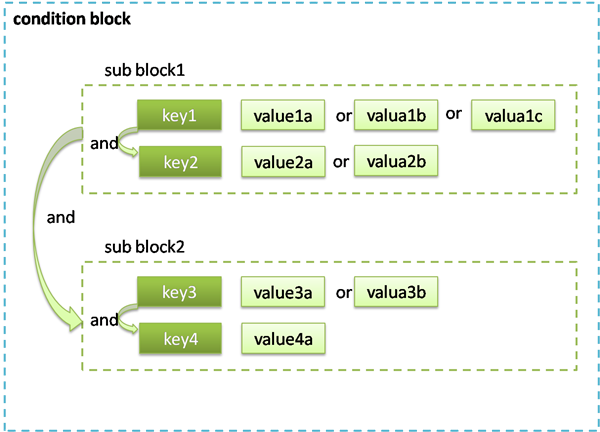# Conditions for Taking Effect

Last updated: 2018-01-08 15:38:51

PDF

### Application Scenarios

In many scenarios, conditions need to be specified for a created policy to take effect.
Scenario 1: When a CAM user calls the cloud API, the source of user access needs to be restricted. It is required to add the IP condition to the existing policy.
Scenario 2: When a CAM user calls the VPC peering connection API, it is required to determine whether the user has the permission to access peering connection API and peering connection resources, as well as the VPC associated with the peering connection.

### Syntax Structure

The syntax structure of condition is shown in the following figure. A condition block consists of multiple sub-blocks. Each sub-block corresponds to a conditional operator and several condition keys. Each condition key corresponds to several condition values.### Evaluation Logic

The evaluation logic that enables a condition to take effect is as follows:

1. A condition key can correspond to multiple condition values. The condition becomes effective only when the key in the context corresponds to any one of these condition values upon execution of the associated conditional operator.

2. If a sub condition block has multiple condition keys, the sub condition becomes effective only when the condition that corresponds to all condition keys takes effect.

3. If a block contains multiple sub condition blocks, the entire condition becomes effective only when each sub condition block takes effect.

4. For a conditional operator ending in _if_exist, the condition takes effect even if the context does not include the condition key associated to the conditional operator.

5. "for_all_value" is a qualifier that restricts the conditional operator, which is applicable to the scenarios where the condition key in the context contains multiple values. The entire condition becomes effective only when each value of the condition key takes effects upon execution of the associated conditional operator.

6. "for_any_value" is a qualifier that restricts the conditional operator, which is applicable to the scenarios where the condition key in the context contains multiple values. The entire condition becomes effective only when any value of the condition key takes effects upon execution of the associated conditional operator.

### Examples

1. The following example shows that a user can only call the cloud API within `10.217.182.3/24` or `111.21.33.72/24` IP address range.
``````{
"version": "2.0",
"statement": {
"effect": "allow",
"action": "cos:PutObject",
"resource": "*",
"condition": {"ip_equal": {"qcs:ip": ["10.217.182.3/24", "111.21.33.72/24"]}}
}
}
``````
2. The following example describes that the region of VPC must be Shanghai when it is allowed to bind to a specified NAT gateway.
``````{
"version": "2.0",
"statement": {
"effect": "allow",
"action": "name/vpc:AcceptVpcPeeringConnection",
"resource": "qcs::vpc:sh::pcx/2341",
"condition": {"string_equal_if_exist": {"vpc:region": "sh"}}
}
}
``````

### Conditional Operator List

The following table provides the information of conditional operators, condition names and examples. For more information about the custom condition key for each product, please see the product documentation.

Conditional Operator Description Condition Name Example
string_equal String is equal to (case-sensitive) qcs:tag {"string_equal":{"qcs:tag/tag_name1":"tag_value1"}}
string_not_equal String is not equal to (case-sensitive) qcs:tag {"string_not_equal":{"qcs:tag/tag_name1":"tag_value1"}}
string_equal_ignore_case String is equal to (case-insensitive) qcs:tag {"string_equal_ignore_case":{"qcs:tag/tag_name1":"tag_value1"}}
string_not_equal_ignore_case String is not equal to (case-insensitive) qcs:tag {"string_not_equal_ignore_case":{"qcs:tag/tag_name1":"tag_value1"}}
string_like String matches (case-sensitive) qcs:tag {"string_like":{"qcs:tag/tag_name1":"tag_value1"}}
string_not_like String does not match (case-sensitive) qcs:tag {"string_not_like":{"qcs:tag/tag_name1":"tag_value1"}}
date_not_equal Date is not equal to qcs:current_time {"date_not_equal":{"qcs:current_time":"2016-06-01T00:01:00Z"}}
date_greater_than Date is greater than qcs:current_time {"date_greater_than":{"qcs:current_time":"2016-06-01T00:01:00Z"}}
date_greater_than_equal Date is greater than or equal to qcs:current_time {"date_greater_than_equal":{"qcs:current_time":"2016-06-01T00:01:00Z"}}
date_less_than Date is less than qcs:current_time {"date_less_than":{"qcs:current_time":"2016-06-01T 00:01:00Z"}}
date_less_than_equal Date is less than or equal to qcs:current_time {"date_less_than":{"qcs:current_time":"2016-06-01T 00:01:00Z"}}
date_less_than_equal Date is less than or equal to qcs:current_time {"date_less_than_equal":{"qcs:current_time":"2016-06-01T00:01:00Z"}}
Ip_equal IP is equal to qcs:ip {"ip_equal":{"qcs:ip ":"10.121.2.10/24"}}
Ip_not_equal IP is not equal to qcs:ip {"ip_not_equal":{"qcs:ip":["10.121.2.10/24","10.121.2.20/24"]}}
numeric_not_equal Valueis not equal to qcs:mfa {"numeric_not_equal":{"mfa":1}}
numeric_greater_than Value is greater than {"numeric_greater_than":{"cvm_system_disk_size":10}}
numeric_greater_than_equal Value is greater than or equal to {"numeric_greater_than_equal":{"cvm_system_disk_size":10}}
numeric_less_than Value is less than {"numeric_less_than":{"cvm_system_disk_size":10}}
numeric_less_than_equal Value is less than or equal to {"numeric_less_than_equal":{"cvm_system_disk_size":10}}
numeric_not_equal Value is equal to qcs:mfa {"numeric_not_equal":{"mfa":1}}
numeric_greater_than Value is greater than {"numeric_greater_than":{"some_key":11}}
bool_equal Boolean matches - -
null_equal Condition key matches empty string - -

Notes:

1. Date is displayed in a format that conforms to the ISO8601 standard, and UTC time is used.

2. The IP format must comply with the CIDR standard.

3. A conditional operator (excluding null_equal) ending in _if_exist indicates that the condition takes effect even if the context does not include the corresponding key value.

4. "for_all_value" is a qualifier that needs to be used with the conditional operator, which means that a condition takes effects when each value of the conditional key in the context meets the requirement of the condition.

5. "for_any_value" is a qualifier that needs to be used with the conditional operator, which means that a condition takes effects when any value of the conditional key in the context meets the requirement of the condition.